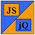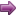# JavaScript & jQuery Tutorials

JavaScript & jQuery Quizzes HomeJavaScript Basics Quiz

## JavaScript Basics Quiz

The questions in this first quiz on JavaScript 1.5 are on the topics covered in the JavaScript Basics section of the site. The table below lists the lessons, a description of the lesson content and the the quiz question number range.

### Lesson Summary

Click on a lesson in the table to go to that lesson for a refresher on the topics for that lesson.

JavaScript 1.5 Basics Lessons Description Question Range
Lesson 1 - Getting StartedIn this lesson we introduce javaScript 1.5.1 - 2
Lesson 2 - JavaScript SyntaxThis lesson is all about JavaScript syntax and how to apply it.3 - 6
Lesson 3 - The Anatomy of JavaScriptIn this lesson we list all the predefined JavaScript globals, statements and operators.7 - 11
Lesson 4 - Applying JavaScriptFor this lesson we look at incorporating JavaScript into our HTML code.12 - 15
Lesson 5 - Basic Maths FunctionsIn this tutorial we learn how to apply basic maths functions in JavaScript.16 - 18
Lesson 6 - VariablesThis lesson is all about JavaScript variables and the naming rules we use with them.19 - 22
Lesson 7 - Objects In this lesson we learn how to create `Object` instances and assign property values to them.23 - 26
Lesson 8 - StringsIn this lesson we learn how to use and manipulate strings and create `String` objects.27 - 30
Lesson 9 - BooleansIn this lesson we learn how to use and manipulate the `Boolean` object.31 - 33

### JavaScript 1.5 Quiz

The quiz below tests your knowledge of the material learnt in the JavaScript Basics section of the site.

Question 1 : What do we use JavaScript for in our web pages?
- We use JavaScript for behaviour, CSS for presentation and HTML for structure.
Next >>
Question 2 : Where do we place our <script></script> HTML element?
- The <code>script</code> HTML tag can be placed in either the <code>head</code> or <code>body</code> HTML tag.
<< Prev
Next >>
Question 3 : JavaScript is a compiled language?
- JavaScript is an interpreted language which leads to faster development but slower runtimes than compiled languages.
<< Prev
Next >>
Question 4 : What are JavaScript Constructors used for?
- We use JavaScript Constructors to create objects or use predefined objects.
<< Prev
Next >>
Question 5 : What do we use to perform repetetive tasks?
- We use functions to perform repetetive tasks.
<< Prev
Next >>
Question 6 : The code for a function is inserted between what symbols?
- The code for a function is inserted between { }. Opening and closing braces.
<< Prev
Next >>
Question 7 : What category do `Nan` and `Infinity` come under?
- <code>Nan</code> and <code>Infinity</code> come under the Property category.
<< Prev
Next >>
Question 8 : We create `Math` objects using a Constructor?
- The <code>Math</code> object is static and as such we cannot create objects of this type.
<< Prev
Next >>
Question 9 : What category does `undefined` come under?
- <code>undefined</code> comes under the Variable category.
<< Prev
Next >>
Question 10 : All predefined objects apart from the static `Math` class have a `constructor` method?
- The <code>JSON</code> object is also static and as such doesn't have a <code>constructor</code> method either.
<< Prev
Next >>
Question 11 : What category do `Boolean` and `Regexp` come under?
- <code>Boolean</code> and <code>Regexp</code> come under the Constructor category.
<< Prev
Next >>
Question 12 : Inline scripting is the most unobtrusive way to code our JavaScript?
- Inline scripting is actually the most obtrusive way to code our JavaScript as it mixes behaviour (JS) with structure (HTML), is prone to error and leads to higher maintenance.
<< Prev
Next >>
Question 13 : Which of the following is the preferred way to use JavaScript to lower maintenance overhead?
- The preferred way to use JavaScript to lower maintenance overhead is by using external JavaScript files as changes can be made in one place.
<< Prev
Next >>
Question 14 : What is the effective number of times we can use the `Window.onload` method in a web page?
- We can use the `Window.onload` method as many times as we like in a web page and whether placed in the <code>head</code> or <code>body</code> elements is irrelelevant. The 'gotcha' is that only the last read in <code>Window.onload</code> is the one used and so this method only really has one effective use.
<< Prev
Next >>
Question 15 : We can use a combination of external JavaScript files, internal JavaScript file and inline scripting in a web page?
- We can use a combination of external JavaScript files, internal JavaScript file and inline scripting in a web page.
<< Prev
Next >>
Question 16 : Division and Multiplication take precedence over Addition and Subtraction when performing several mathematical operations in the same statement?
- Division and Multiplication do take precedence over Addition and Subtraction when performing several mathematical operations in the same statement.
<< Prev
Next >>
Question 17 : What symbols do we use to group mathematical operations we want performed first.?
- We use parentheses to group mathematical operations we want performed first..
<< Prev
Next >>
Question 18 : What is the following code an example of?
`aTotal += 10`
- The code is an example of a mathematical shortcut.
<< Prev
Next >>
Question 19 : Which symbol can we use to create multiple variables at the same time by delimiting the end of each variable name?
- You can create multiple variables at the same time by delimiting the end of each variable name with the comma (,) symbol.
<< Prev
Next >>
Question 20 : Which of the following is an invalid name for a variable?
- Only letters, the dollar (\$) and underscore (_) symbols are allowed as the first character of the variable name.
<< Prev
Next >>
Question 21 : After variable creation you can store data of any type within it by using the equals symbol (=) via assignment.?
- JavaScript is not a 'strongly typed' language so after variable creation you can store data of any type within it by using the equals symbol (=) via assignment..
<< Prev
Next >>
Question 22 : Which of the following is a valid name for a variable?
- Only letters, numbers, the dollar (\$) and underscore (_) symbols are allowed when creating variable names.
<< Prev
Next >>
Question 23 : Which special operator do we use to create `Object` instances?
- We use the <code>new</code> special operator to create <code>Object</code> instances?.
<< Prev
Next >>
Question 24 : How do we create `Object` instance properties?
- We create <code>Object</code> instances through assignment.
<< Prev
Next >>
Question 25 : What happens when we try to display an `Object` instance property that doesn't exist?
- When we try to display an <code>Object</code> instance property that doesn't exist, the property is returned as <code>undefined</code>.
<< Prev
Next >>
Question 26 : How can we get rid of unwanted properties of an `Object` instance?
- We can get rid of unwanted properties of an <code>Object</code> instance using the <code>delete</code> special operator.
<< Prev
Next >>
Question 27 : What is the following code an example of?
`bString = 'isThisValid'; `
- <code>bString = 'isThisValid';</code> is an example of a 'string primitive'.
<< Prev
Next >>
Question 28 : What is the disadvantage of using string literals compared to using a `String` instance?
- There are no disadvantages to using string literals as JavaScript converts any strings to temporary <code>String</code> objects when a <code>String</code> method is called on the string.
<< Prev
Next >>
Question 29 : What symbol do we use when concatenating strings together?
- We use the <code>+</code> (plus) symbol when concatenating strings together.
<< Prev
Next >>
Question 30 : What value is alerted from the following code?
`var aString = '5';var aNumber = 5;alert(aString + aNumber);`
- The value <code>55</code> is alerted from the code as all numbers are converted to strings before concatenation takes place.
<< Prev
Next >>
Question 31 : What is alerted from the following code?
```aBoolean = new Boolean(false); if (aBoolean) { alert('false'); } else { alert('true'); }```
- When using a <code>Boolean</code> object any values other than <code>undefined</code> and <code>null</code> evaluate to <code>true</code> when passed to a conditional statement.
<< Prev
Next >>
Question 32 : What is alerted from the following code?
```aBoolean = false; if (aBoolean) { alert('false'); } else { alert('true'); }```
- When using boolean primitives the logic works as expected and the value <code>false</code> is returned.
<< Prev
Next >>
Question 33 : What should we use for conditional statements?
- We should use boolean primitives for conditional statements and the <code>Boolean</code> constructor when we just want to wrap a boolean value.
<< Prev
Finish!
Quiz Progress Bar Please select an answer

### What's Next?

In the next quiz we test our knowledge of the topics covered in the JavaScript Intermediate section of the site .